# Maharashtra Board Class 11 Economics Important Questions Chapter 3 Partition Values

Balbharti Maharashtra State Board Class 11 Economics Important Questions Chapter 3 Partition Values Important Questions and Answers.

## Maharashtra State Board 11th Economics Important Questions Chapter 3 Partition Values

1A. Complete the correlation:

Question 1.
Quartiles : 4 parts : : 10 parts : __________
Deciles

Question 2.
10 parts : Deciles : : Percentiles : __________
100 partsQuestion 3.
Individual Data : __________ : : Discrete Data : $$\bar{x}=\frac{\sum f_{i} x_{i}}{n}$$
$$\bar{x}=\frac{\sum x}{n}$$

Question 4.
Q2 : Q50 : : D5 : __________
Median

1B. Suggest the economic terms for the given statements:

Question 1.
The value that divides the data into hundred equal parts.
Percentiles

Question 2.
Values that divide the data into the required number of equal parts.
Partition values or Fractiles

Question 3.
Value of the middlemost observation in the data.
Median

Question 4.
Extreme value on the lower side and higher side in magnitude.
OutliersQuestion 5.
Q2 = D5 = P60
Median

Question 6.
Partition value used in the measurement of test scores, health indicators, etc.
Percentiles

Question 7.
Partition value is used to assess the performance of portfolio investment.
Declies

1C. Arrange in proper order:

Question 1.
Steps to find Q1 and Q3 for continuous frequency distribution.
(a) Determine the quartile class
(b) Arrange the data in ascending or descending order.
(c) Find out cumulative frequency (cf)
(d) Write respective frequencies of the class
Options:
(1) a, b, c, d
(2) b, d, c, a
(3) b, c, d, a
(4) c, d, b, a
(1) b, d, c, a

1D. Find the odd word out:

Question 1.
Percentile, Mercantile, Decile, Quartile
Mercantile

Question 2.
Mean, Mode, Meridian, Median
MeridianQuestion 3.
P.C.Mahalanobis, Statistics Day, Fractile Graphical Analysis, Demand Analysis.
Demand Analysis

Question 4.
Percentile, Health indicator, Quartile, Household income.
Quartile

1F. Complete the following statement.

Question 1.
There are __________ deciles.
nine

Question 2.
There are __________ percentiles.
ninety-nine

Question 3.
There are __________ quartiles.
three

Question 4.
50th percentile is equal to __________ deciles.
fifthQuestion 5.
25th percentile is equal to __________ quartile.
first

Question 6.
Percentiles divide the series in __________ equal parts.
hundred

Question 7.
Value that divides the data into 10 equal parts is called __________
deciles

Question 8.
50th percentile is equal to __________
5th decile

Question 9.
The value of the first quartile is __________
$$\left(\frac{n+1}{4}\right)^{\text {th }}$$ observation

Question 10.
The value of the 3rd quartile is __________
$$3\left(\frac{n+1}{4}\right)^{\text {th } \text { Observation }}$$

Question 11.
Value that divides the whole set of observation into four equal parts is called __________
QuartileQuestion 12.
29th June is celebrated as __________
Statistics Day

Question 13.
Value which divide the data into required number of equal parts are called __________
partition values

Question 14.
Fractile graphical analysis, a statistical method was devised by __________
Prof. P. C. Mahalanobis.

2. Distinguish between:

Question 1.
Quartiles and Deciles.

 Quartiles Deciles (i) Quartiles are the values that divide the series into four equal parts. (i) Deciles are the values that divide the series into ten equal parts. (ii) These are the 3 values that divide the distribution into 4 parts, each representing one-quarter of the score. (ii) These are the 9 values that divide the distribution into 10 parts. (iii) It is denoted by: Q1, Q2, and Q3 (iii) It is denoted by D1, D2, D3, …………. D9 (iv) 2nd Quartile is the median. (iv) 5th Decile is the median.

Question 2.
Deciles and Percentiles.

 Deciles Percentiles (i) Deciles are the values that divide the series into 10 equal parts. (i) Percentiles are the values that divide the series into 100 equal parts. (ii) These are the 9 values that divide the distribution into 10 parts. (ii) These are the 99 values that divide the distribution into 100 parts. (iii) It is denoted by D1, D2, D3, ……. D9 (iii) It is denoted by P1, P2, P3, ……… P99 (iv) 5th Decile is median. (iv) 50th percentile is median.

3. Solve the following:

Type – I: Problems Based on Quartiles

Question 1.
Find Q1, Q2 and Q3 from the following data:
65, 82, 68, 67, 84, 83, 66, 69, 81, 70, 85
Q1 = 67, Q2 = 70, Q3 = 83Question 2.
Find Q1 and Q3 from the following data:
3, 5, 6, 7, 8, 9, 10, 11, 12, 13, 15
Q1 = 6, Q3 = 12

Question 3.
Find first and third Quartiles from the following data: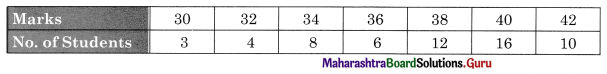Q1 = 34, Q3 = 40

Question 4.
Find Q1, Q2, and Q3?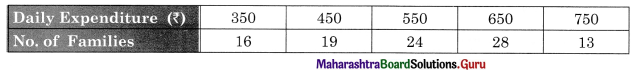Q1 = 450, Q2 = 550, Q3 = 650

Question 5.
Find Q1 and Q3 from the following data: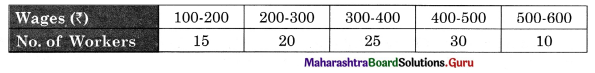Q1 = 250, Q3 = 450

Question 6.
Find Q4 and Q3 from the following data: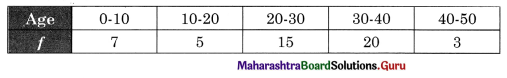Q4 = 20.33 , Q3 = 35.25Question 7.
Find Q1, Q2, and Q3: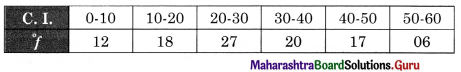Q1 = 17.22, Q2 = 27.41, Q3 = 39

Type – II: Problems Based on Decile

Question 1.
Find D4 and D8 from the following data:
20, 26, 24, 32, 22, 28, 30, 36, 34
D4 = 26, D8 = 34

Question 2.
Find D1, D4, and D8 for the following data:
61, 62, 63, 64, 65, 66, 67, 68, 69, 70, 71, 72, 73, 74, 75, 76, 77, 78, 79.
D1 = 62, D4 = 68, D8 = 76

Question 3.
Find D2 and D4 from the following data: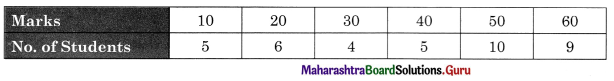D2 = 20, D4 = 40

Question 4.
Find D5 and D9: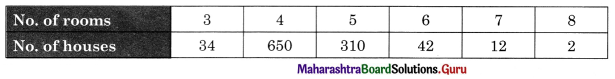D5 = 4, D9 = 5Question 5.
Find D5 for the following distribution.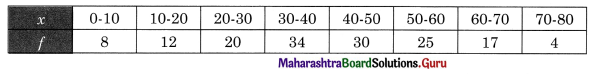D5 = 40.33

Question 6.
Find D4 and D6 from the following data: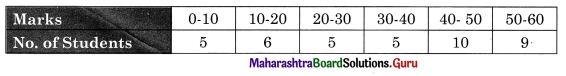D4 = 30, D6 = 43

Type – III: Problems Based on Percentiles

Question 1.
Find Percentiles:
Calculate 50th Percentile from the following data:
20, 25, 30, 35, 40, 45, 50, 55, 60
P50 = 40

Question 2.
Calculate P21 and P97 for: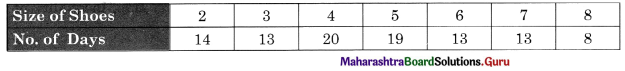P21 = 3, P97 = 8Question 3.
Find P90 from the following data: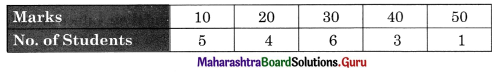P90 = 40

Question 4.
Calculate P60 and P80 from the following data: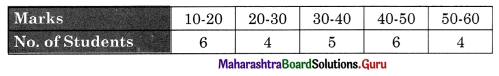P60 = 40, P80 = 48.33

Type – IV: Comprehensive Problems

Question 1.
Find Q1, D5 and P45 for the following data: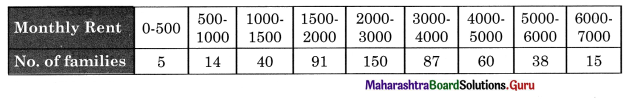Q1 = 1862.64, D5 = 2666.67, P45 = 2500

4. State with reasons whether you agree or disagree with the following statements

Question 1.
Q2 divides the series into equal parts.
Yes, I agree with the statement.

• Quartiles are the values of data that divide the series or data into four equal parts.
• Each represents one-quarter of the data when arranged in ascending and descending order.
• These 3 values are called Q1, Q2, and Q3.
• Q2 is nothing but the median or the middlemost value.
• Hence, Q2 divides the series into two equal parts.Question 2.
The 25th percentile is equal to the first quartile.